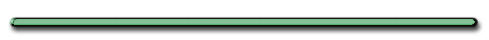Rank the Vertical Velocity from Largest to smallest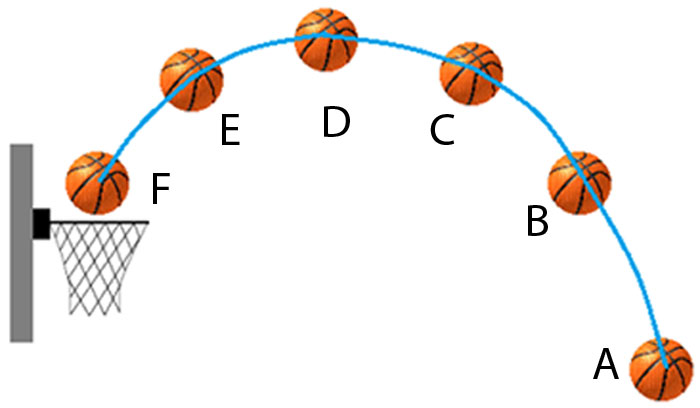Rank the horizontal displacement from least to greatest.Explain.

The football has 2 velocities.
Which velocity is the football player matching.

[ Flash ]

Show Me The Physics

Horizontal velocity.
The football and the football player
have a constant horizontal velocity.

Show Me The Physics

 Ex) A projectile leaves ground at an angle of 60.° and a speed of 100.m/s. (a) Find the initial vertical component of the object's velocity

Ex) A projectile leaves ground at an angle of 60.° and a speed of  100. m/s.

(a) Find the initial vertical component of the object's velocity

Ө = 60.°

Vi  = 100. m/s
Viy = ?Viy = Vi(sinӨ)

= (100. m/s)sin60.°

Viy = 86.6 m/s up

(b) Find the objects maximum height.

Viy = 86.6 m/s
ay = -9.81 m/s2 (ascending)
dy (max) =?
Vfy = 0 (at max height)

02 = (86.6 m/s)2 + 2(-9.8 m/s2)d

0 = 7499 + -19.6.dy

subtract 7499 from both sides

-7499 = -19.6(dy)

dy = 382 m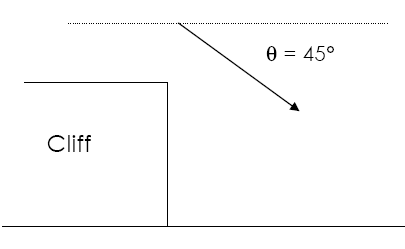ex) A rock is thrown from a cliff with initial speed of 40. m/s at an angle of 45.° below  the horizontal.  (a) What is the vertical component of the initial velocity?
`        `

a) What is the vertical component  of the initial velocity?Ө = 45.°

` `
`Vi = 40. m/s`
`Viy = ?     `
` `
`Viy = VsinӨ`
` `
`= (40. m/s)sin45.° `

` `
`Viy = 28 m/s down `

(b) If the rock strikes ground in 1 sec what is height of cliff?

(b) If the rock strikes ground in 1.0 sec what is height of cliff?

dy = ? (height)

ay = +9.8 m/s2

t = 1.0 sec

Viy = 28 m/s (from before)

`dy = Viyt + (½)at2`
` `

= 28.m/s(1.0sec)+½(9.81 m/s2)(1.0sec)2

`28 m + 4.9 m`
` `

` `
`dy = 32.9 m down`
` `
` `
` `
`dy = 33 m down`
` `
` `
` `
` `
` `
` `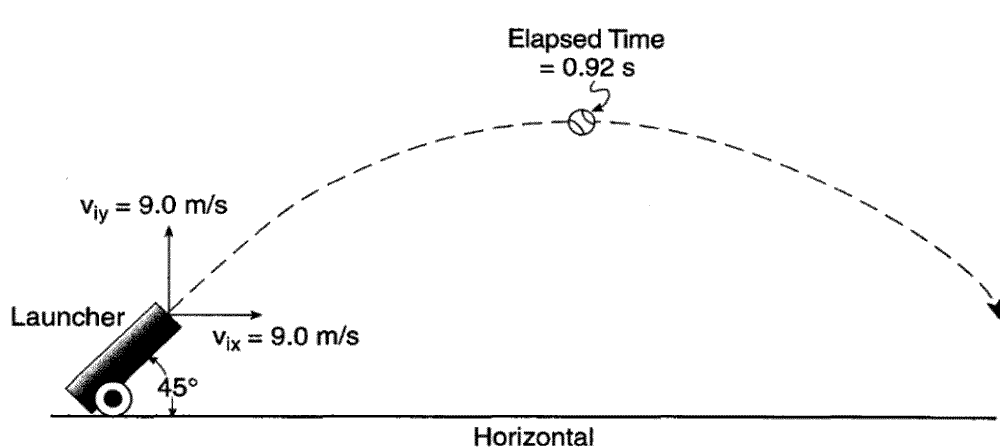At Peak,

Vx = ?Vx = 9.0 m/s

Total Time in Air?1.84 sec
(twice peak)

actually 1.8

(2 sig. figs.)What will the horizontal velocity of the baseball be just before it hits the ground?

9 m/s, Vx is constant[ HTML5 ]
Don Ion

(offsite)Flash Physics - Don Ion| Onsite |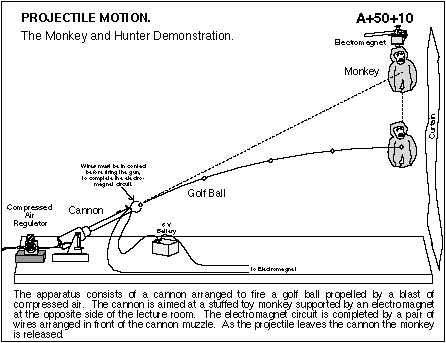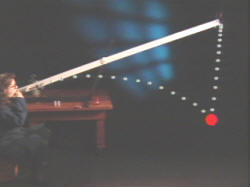Projectile Motion Game
Show Me The Physics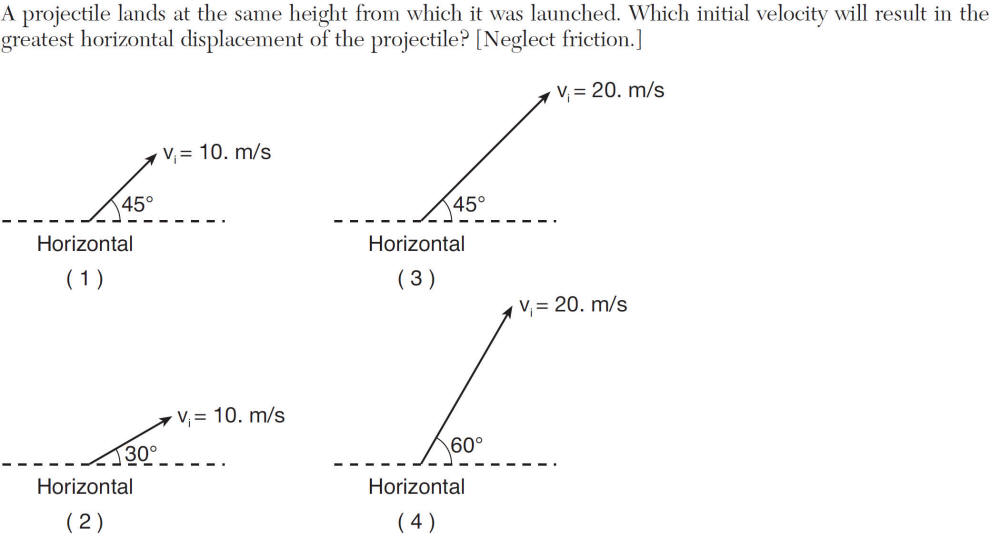Choice 3